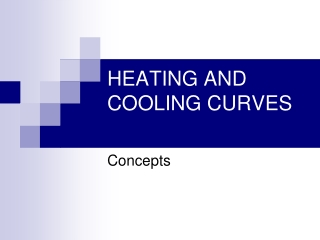DownloadDownload PresentationHEATING AND COOLING CURVES

# HEATING AND COOLING CURVES

Download Presentation## HEATING AND COOLING CURVES

- - - - - - - - - - - - - - - - - - - - - - - - - - - E N D - - - - - - - - - - - - - - - - - - - - - - - - - - -
##### Presentation Transcript

1. HEATING AND COOLING CURVES Concepts

2. What are some things that happen as we heat a sample up? • Solid  Liquid  Gas • Melting, Evaporating • Increase in entropy

3. What is heat? How is heat different from temperature? • Heat is a measure of kinetic energy • In chemistry heat is measured in Joules (J) • Heat is dependent on mass, temperature change and specific heat • Temperature is a measure of average kinetic energy • Temperature is measured in Kelvin (K) or Celsius (C)

4. What is the heating curve? • The heating curve is a graph which represents how a sample changes phases. As heat is added over time, the sample changes temperature and phase accordingly. Thus heating curve.

5. How does the heating curve look?

6. What are the parts of the heating curve?

7. What are the parts of the heating curve?

8. What are the parts of the heating curve?

9. What are the parts of the heating curve?

10. What are the parts of the heating curve?

11. What are the parts of the heating curve?

12. Why is the curve flat at some portions? • Temperature is staying constant, but potential energy is increasing • During this time the solid completely becomes liquid

13. Why is the curve flat at some points? • This is called the heat of fusion (Hf) in segment BC and heat of vaporization (Hv) in segment DE. • Remember fusion is melting and vaporization is evaporation

14. What is heat of fusion? What is heat of vaporization? • Hf is the amount of energy needed to completely make a solid into a liquid • Hv is the amount of energy needed to completely make a liquid into a gas

15. Summary • The heating curve is a useful tool to show us the changes in temperature, energy, and entropy as a sample is heated up. It give us detailed information about phases and phase changes of samples.